# Community

Blog Events Webinars Tutorials Forum
×
Community Blog A Deep Analysis of Virtual Keywords

# A Deep Analysis of Virtual KeywordsBy Lin Shaohua (Yijue)

## Introduction

What made me write this article? Virtual does not have any superpowers. Everything exist for a reason, but it is undesirably wrong to abuse it. This article will walk you through how virtual works and unveil its mystery.

## Why Do We Need Virtual?

Let’s say we are working on the design and implementation of a public graphical library, which involves printing 2D and 3D coordinate points. The implementation of Point2D and Point3D is designed below:

``````#include <stdio.h>
class Point2d {
public:
Point2d(int x = 0, int y = 0): _x(x), _y(y) {}
void print() const { printf("Point2d(%d, %d)\n", _x, _y); }
protected:
int _x;
int _y;
};
class Point3d : public Point2d {
public:
Point3d(int x = 0, int y = 0, int z = 0):Point2d(x, y), _z(z) {}
void print() const { printf("Point3d(%d, %d, %d)\n", _x, _y, _z); }
protected:
int _z;
};
int main() {
Point2d point2d;
Point3d point3d;
point2d.print();        //outputs: Point2d(0, 0)
point3d.print();        //outputs: Point3d(0, 0, 0)
return 0;
}``````

Perfect. Everything works as expected. In that case, why do we need virtual? Let's make a new requirement: encapsulate the printing interface of a coordinate point, in which the input is the coordinate point instance, and the output is the value of the coordinate point. Soon, the code is implemented:

``````void print(const Point2d &point) {
point.print();
}
int main() {
Point2d point2d;
Point3d point3d;
print(point2d);       //outputs: Point2d(0, 0)
print(point3d);       //outputs: Point2d(0, 0)
return 0;
}``````

Here is the problem. When we pass in a 3D coordinate point instance, we want to print the value of the 3D coordinate point, but only the value of the 2D coordinate point can be printed. The current program can't tell whether the coordinate point is 2D or 3D. Virtual emerges to make the program smart enough to tell the difference. You only need to update the declaration of the Point2D interface print:

``````class Point2d {
public:
virtual void print() const { printf("Point2d(%d, %d)\n", _x, _y); }
};
int main() {
Point2d point2d;
Point3d point3d;
print(point2d);       //outputs: Point2d(0, 0)
print(point3d);       //outputs: Point3d(0, 0, 0)
return 0;
}``````

Well done. Everything is back to normal. Virtual is needed to realize the power of polymorphism in C++ inheritance. What makes virtual so magical? It all starts with the memory layout of class data members.

## The Memory Layout of the Class

In the C++ object model, non-static data members are configured within each class object, and static data members are stored outside the class object. Static and non-static function members are also stored outside the class object. Most compilers arrange the memory layout of classes in the order that members are declared. All examples in this article are compiled using x86_64-apple-darwin21.6.0/clang-1300.0.29.3 in the Mac environment, with a Point2D memory layout of the non-virtual version: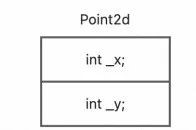Memory layout needs us to pay attention to the alignment of memory by the compiler. Memory alignment is generally divided into two steps. The first step is that class members are aligned according to their size. The second step is that classes are aligned according to the maximum size of their members. When we arrange class members, we should follow the declaration of members from the largest to the smallest, which can avoid unnecessary memory filling and save memory usage.

## Memory Layout of Derived Classes

In the C++ inheritance model, the memory size of a subclass is the sum of the size of its base classes' data members plus its data members. Most compilers arrange the data members of the base class and then the data members themselves for the memory layout of subclasses. The Point3D Memory Layout of the Non-Virtual Version is shown below: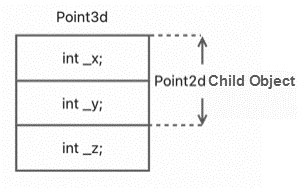## Memory Layout of the Virtual Class

When Point2D declares the virtual function, it has two major effects on the class object. First, the class will generate a series of pointers to virtual functions and put them in a table called a virtual table (vtbl). Second, class instances are all inserted with a pointer to the relevant virtual table. Usually, this pointer is called vptr. We redesigned the Point2D and Point3D implementations for better example results:

``````class Point2d {
public:
Point2d(int x = 0, int y = 0): _x(x), _y(y) {}
virtual void print() const { printf("Point2d(%d, %d)\n", _x, _y); }
virtual int z() const { printf("Point2d get z: 0\n"); return 0; }
virtual void z(int z) { printf("Point2d set z: %d\n", z); }
protected:
int _x;
int _y;
};
class Point3d : public Point2d {
public:
Point3d(int x = 0, int y = 0, int z = 0):Point2d(x, y), _z(z) {}
void print() const { printf("Point3d(%d, %d, %d)\n", _x, _y, _z); }
int z() const { printf("Point3d get z: %d\n", _z); return _z; }
void z(int z) { printf("Point3d set z: %d\n", z); _z = z; }
protected:
int _z;
};``````

Most compilers insert vptr at the beginning of the class instance. Now, let's a look at the Point2D and Point3D memory layout of the virtual version: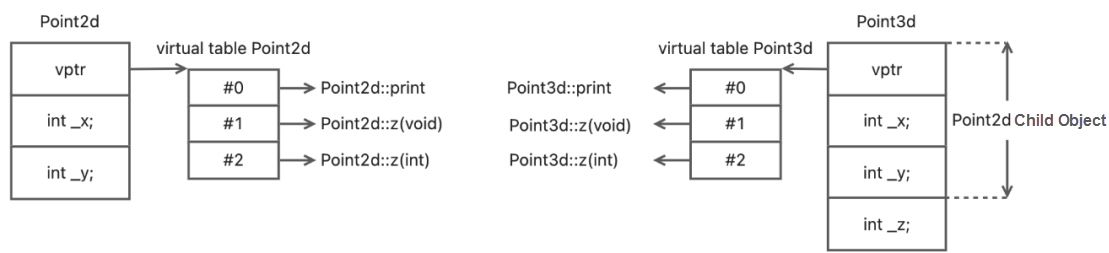As to whether the real memory layout is exactly what is shown in the figure above, we can get the answer through verification:

``````int main() {
typedef void (*VF1) (Point2d*);
typedef void (*VF2) (Point2d*, int);
Point2d point2d(11, 22);
intptr_t *vtbl2d = (intptr_t*)*(intptr_t*)&point2d;
((VF1)vtbl2d)(&point2d);       //outputs: Point2d(11, 22)
((VF1)vtbl2d)(&point2d);       //outputs: Point2d get z: 0
((VF2)vtbl2d)(&point2d, 33);   //outputs: Point2d set z: 33
Point3d point3d(44, 55, 66);
intptr_t *vtbl3d = (intptr_t*)*(intptr_t*)&point3d;
((VF1)vtbl3d)(&point3d);       //outputs: Point3d(44, 55, 66)
((VF1)vtbl3d)(&point3d);       //outputs: Point3d get z: 66
((VF2)vtbl3d)(&point3d, 77);   //outputs: Point3d set z: 77
return 0;
}``````

The acquisition of the virtual table is in line 5, which can be regarded as a two-step operation: intptr_t vptr2d = (intptr_t)&point2d;intptr_t vtbl2d = (intptr_t)vptr2d. The first step is to make the vptr2d point to the virtual table, and the second step is to convert the pointer to the first address of the array. Then, you can use vtbl2d to call virtual functions one by one. From the output result, the program calls the corresponding virtual functions one by one, and the memory layout of the virtual class is consistent with the structure diagram we drew earlier.

What's interesting about it is the definition of the virtual function pointer. Does it remind you of anything? Yes, it is the existence of the C++ class pointer: this pointer in the class member function exists when the compiler passes in the address of the class instance as the first parameter. Like any other parameter, this pointer has nothing special.

## Virtual Destructor

We didn't talk about the destructor above because we want to explain it here. Let's redesign the inheritance system and add the Point class:

``````class Point {
public:
~Point() { printf("~Point\n"); }
};
class Point2d : public Point {
public:
~Point2d() { printf("~Point2d"); }
};
class Point3d : public Point2d {
public:
~Point3d() { printf("~Point3d"); }
};
int main() {
Point *p1 = new Point();
Point *p2 = new Point2d();
Point2d *p3 = new Point2d();
Point2d *p4 = new Point3d();
Point3d *p5 = new Point3d();
delete p1;      //outputs: ~Point
delete p2;      //outputs: ~Point
delete p3;      //outputs: ~Point2d~Point
delete p4;      //outputs: ~Point2d~Point
delete p5;      //outputs: ~Point3d~Point2d~Point
return 0;
}``````

As you can see, in the non-virtual destructor version, the factor that determines the call of the destructor chain in the inheritance system is the declaration type of the pointer. The destructor calls its parent class destructor, starting from the class that declares the pointer type. Now let's declare the destructor of Point as virtual and look at the result of the same call:

``````// Except that the Point destructor is declared virtual, the rest remains unchanged.
int main() {
Point *p1 = new Point();
Point *p2 = new Point2d();
Point2d *p3 = new Point2d();
Point2d *p4 = new Point3d();
Point3d *p5 = new Point3d();
delete p1;      //outputs: ~Point
delete p2;      //outputs: ~Point2d~Point
delete p3;      //outputs: ~Point2d~Point
delete p4;      //outputs: ~Point3d~Point2d~Point
delete p5;      //outputs: ~Point3d~Point2d~Point
return 0;
}``````

In the virtual destructor version, the factor that determines the destructor chain call in the inheritance system is the type of pointer. The destructor calls its parent destructor, starting from the actual type class pointed to by the pointer.

## When Do We Need Virtual?

A large number of classes for some codes of many modules in the project declare destructors as virtual. The point is that such a class is neither designed for base class inheritance nor polymorphic capabilities. Can you understand why it is wrong to abuse virtual? The introduction of virtual is not a wise choice when it is unnecessary. It will bring two obvious side effects: one is the additional pointer-sized memory footprint for each class, and the other is the indirectness of function calls it causes. These two features bring about a double consumption of memory and performance.

The memory consumption is a fixed pointer size, which seems insignificant, but when the class has no members or few members, it will cause more than 100% memory expansion. The consumption of performance is more well-hidden, and virtual will bring about the forced synthesis of constructors, which may be out of many people's expectations. Why? The virtual table pointer needs to be placed properly. So, the compiler needs to do this work when constructing the class. If we declare another virtual destructor, it will introduce another unnecessary composition function, resulting in a double kill of performance. Let's look at the consequences of this:

``````#include <stdio.h>
#include <time.h>

struct Point2d {
int _x, _y;
};
struct VPoint2d {
virtual ~VPoint2d() {}
int _x, _y;
};

template <typename T>
T sum(const T &a, const T &b) {
T result;
result._x = a._x + b._x;
result._y = a._y + b._y;
return result;
}

template <typename T>
void test(int times) {
clock_t t1 = clock();
for (int i = 0; i < times; ++i) {
sum(T(), T());
}
clock_t t2 = clock();
printf("clocks: %lu\n", t2 - t1);
}

int main() {
test<Point2d>(1000000);
test<VPoint2d>(1000000);
return 0;
}``````

Let’s assume the code above is saved as demo.cpp, and the code is compiled into demo using clang++ -o demo demo.cpp. Now, we use nm demo|grep Point2d to view all relevant symbols: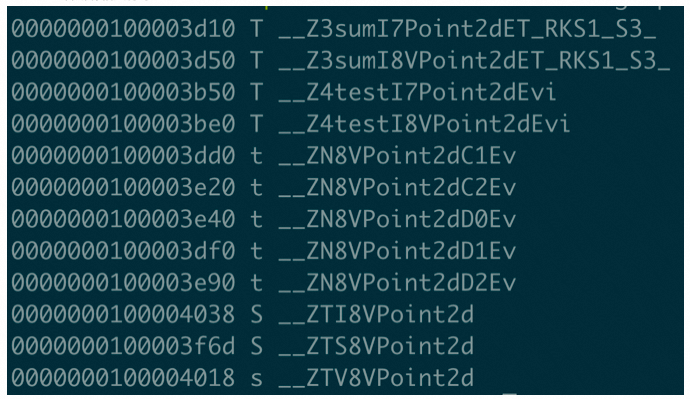You can see that VPoint2D automatically synthesizes the construction, the destructor, and the typeinfo information. By contrast, there is no synthesis of any function for Point2D. Let's look at the execution efficiency of the two: in the macOS environment, the intermediate values of the results of the three demo executions are Point2D:12819 and VPoint2D:21833, and the performance time of VPoint2D is increased by 9014 clocks, an increase of 70.32%. Therefore, you must not introduce virtual at will unless you really need it:

1. When we use polymorphism in inheritance, the virtual functions mechanism is required.
2. When the base class pointer points to a subclass instance, we need to use the virtual destructor.

At any other time, virtual does not have any magic you want, and it will even have a backlash. However, there is another situation in which virtual is needed, virtual base class. Since this situation is too complicated, it is not recommended to try it at any time. (Another long article may be needed to explain more, so no details will be explained here.)

## Summary

This article is an explanation about virtual. We hope it can be helpful and offer readers more understanding. C++ is complex and huge, and there are applicable scenarios and limitations for many of its features. Only by deeply understanding the mechanism behind it can we really learn how to use it properly.

Finally, this article refers to the book entitled Inside the C++ Object Model. Needless to say, I think this is a must-read book about C++. I hope everyone can read it. It will benefit you a lot.

0 1 0
Share on

# Related Products

• ##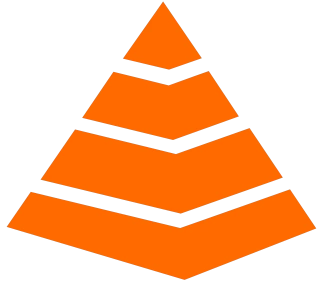Tair

Tair is a Redis-compatible in-memory database service that provides a variety of data structures and enterprise-level capabilities.

• ##VPC

A virtual private cloud service that provides an isolated cloud network to operate resources in a secure environment.

• ##Web Hosting

Explore how our Web Hosting solutions help small and medium sized companies power their websites and online businesses.